# Difference between revisions of "List of problems in mathematical notation"

Overloading/abuse of notation This happens when the same symbol is used for different purposes. For instance, parentheses are used to group expressions, for writing tuples, for function calls, in superscripts or subscripts with various meanings ($f^{(i)}$ for the$i$th derivative or the$i$th function in some sequence), and so forth. The equals sign is often used for equality both in the object language and the metalanguage in mathematical logic, and used as part of the expression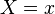$X=x$ to define an event in probability. 0 in linear algebra to mean the scalar 0 as well as the 0 vector of any dimension.
Omission of index Writing things like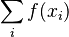$\sum_i f(x_i)$ (unclear what set the index ranges over, or the order in which the terms are added, which can sometimes matter) or even just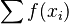$\sum f(x_i)$ (unclear which variable is the index).
Ambiguous order of operations e.g.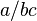$a/bc$
Undefined operations e.g. I often see in mathematical logic things like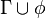$\Gamma \cup \phi$ to mean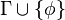$\Gamma \cup \{\phi\}$, where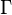$\Gamma$ is a set of formulas and$\phi$ is a formula.